Courses

# Test: Data Handling - 1

## 10 Questions MCQ Test Mathematics (Maths) for Class 3 | Test: Data Handling - 1

Description
This mock test of Test: Data Handling - 1 for Class 3 helps you for every Class 3 entrance exam. This contains 10 Multiple Choice Questions for Class 3 Test: Data Handling - 1 (mcq) to study with solutions a complete question bank. The solved questions answers in this Test: Data Handling - 1 quiz give you a good mix of easy questions and tough questions. Class 3 students definitely take this Test: Data Handling - 1 exercise for a better result in the exam. You can find other Test: Data Handling - 1 extra questions, long questions & short questions for Class 3 on EduRev as well by searching above.
QUESTION: 1

### DIRECTION: Sonam went to a bird sanctuary and saw the different types of birds. Given pictograph shows the number of birds she saw. Study the pictograph and answer the questions.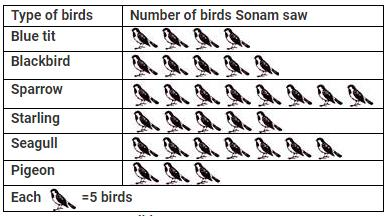Q. How many sparrows did Sonam saw?

Solution:

Number of sparrows seen by Sonam = 8 × 5 = 40

QUESTION: 2

### DIRECTION: Sonam went to a bird sanctuary and saw the different types of birds. Given pictograph shows the number of birds she saw. Study the pictograph and answer the questions.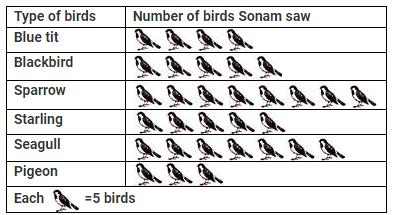Q. How many blue tits and pigeons were seen by Sonam?

Solution:

Number of blue tits seen by Sonam = 4×5=20
∴ Number of pigeons seen by Sonam = 3×5=15
∴Total number of blue tits and pigeons seen by Sonam = 20+15 = 35

QUESTION: 3

### DIRECTION: Sonam went to a bird sanctuary and saw the different types of birds. Given pictograph shows the number of birds she saw. Study the pictograph and answer the questions.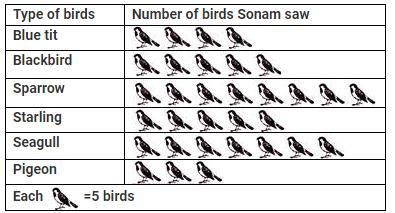Q. How many more seagulls were seen by Sonam than the blackbirds?

Solution:

Number of seagulls seen by Sonam       = 7 × 5 =35
Number of blackbirds seen by Sonam      = 5 × 5 = 25
∴ Required difference = 35−25=10 Hence, 10 more seagulls than blackbirds were seen by Sonam.

QUESTION: 4

DIRECTION: Sonam went to a bird sanctuary and saw the different types of birds. Given pictograph shows the number of birds she saw. Study the pictograph and answer the questions.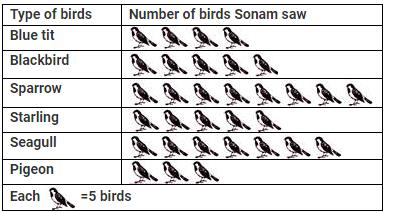Q. How many starlings were seen by Sonam?

Solution:

Number of starlings seen by Sonam  = 5 × 5 = 25

QUESTION: 5

DIRECTION (5-7): The given pictograph shows the number of students who were absent in a day of a particular week. Study the pictograph and answer the following questions.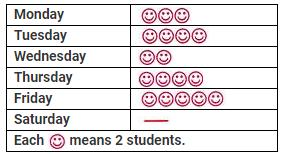Q. On which day no student was absent?

Solution:

On Saturday, no student was absent.

QUESTION: 6

On which day maximum number of students were absent and how many?

Solution:

Maximum number of students were absent on Friday. Number of students absent on Friday  = 5 × 2 = 10

QUESTION: 7

What is the total number of students who were absent in the week?

Solution: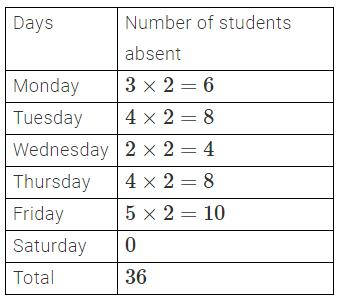QUESTION: 8

Five friends made a graph of the number of marks they got in mathematics.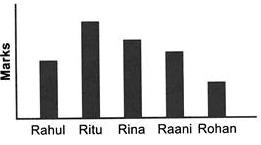Arrange the marks of the students in ascending order?

Solution:

According to the bar graph, the marks of friends in ascending order are as follows: Rohan, Rahul, Raani, Rina, Ritu.

QUESTION: 9

DIRECTION: The given bar graph shows the time taken by 6 competitors to run a 200 m race. Study the given graph and answer the following questions.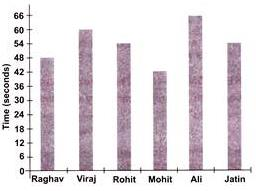Q. Who completed the race before Ali but after Jatin?

Solution:

Viraj completed the race after Jatin but before Ali.

QUESTION: 10

DIRECTION: The given bar graph shows the time taken by 6 competitors to run a 200 m race. Study the given graph and answer the following questions.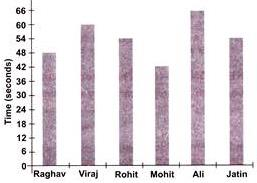Q. Who took time more than 42 seconds but less than 50 seconds?

Solution:

Raghav take 48 seconds which is more than 42 seconds but less than 50 seconds.